# Types Of Angles - Definition with Examples

The Complete K-5 Math Learning Program Built for Your Child

• 40 Million Kids

Loved by kids and parent worldwide

• 50,000 Schools

Trusted by teachers across schools

• Comprehensive Curriculum

Aligned to Common Core

## Types of Angles

Angle

If two lines meet at a point, then the angle between them is the amount of turn, in degrees, that is needed around the point of intersection, to make the lines the same.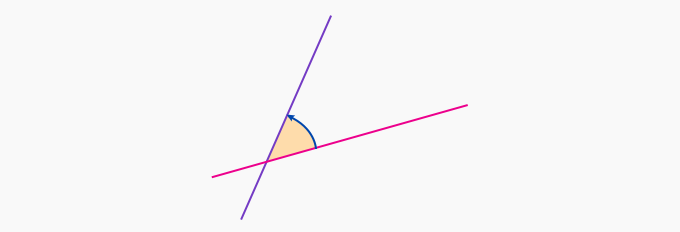Angles are measured in degrees, a complete whole turn is 360º as shown.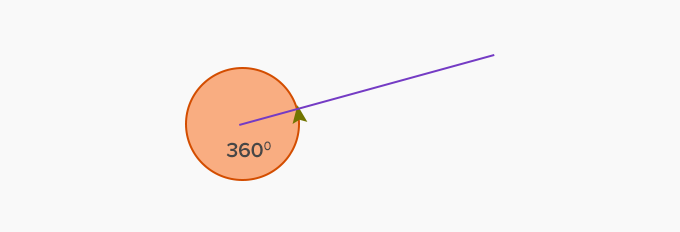Different types of angles

Straight angle: It is one-half of a whole turn, and is the same as the angle made by rays going in opposite directions. The measure of the angle is 180º. Some examples are shown below: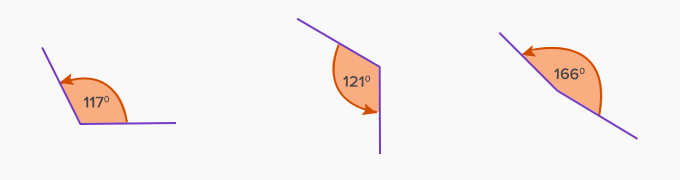Right angle: It is one-quarter of a whole turn and is the same as the angle made by a horizontal line and vertical line. The measure of the angle is 90º. Some examples are shown below: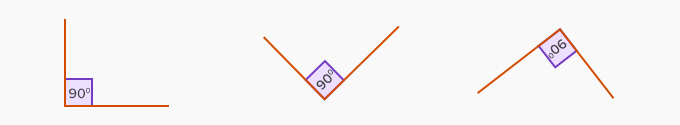Acute angles: The angles that lie between 0º and 90º are called acute. Some examples are shown below: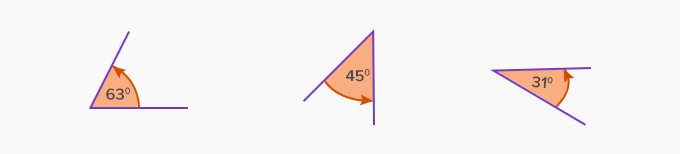Obtuse angles: The angles that lie between 90º and 180º are called obtuse. Some examples are shown below:Reflex angles: The angles that lie between a straight angle (180º) and a whole turn (360º) are called reflex angles. Some examples are shown below: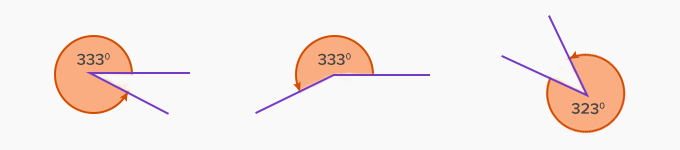## Application of angles

We see angles almost everywhere in our day to day life. For example, the angle made by the hands of a clock, the angle made by two intersecting roads, and angles made by blades of a fan. We also use angles for navigation of planes and ships.

 Fun Facts Angulus is the Latin word from which the word angle is derived.

Won Numerous Awards & Honors